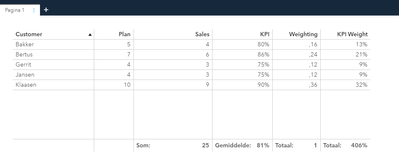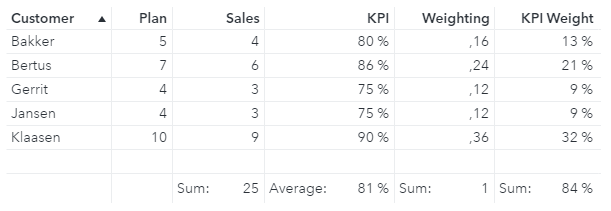## [VA] - tabel, calculations. how to solve

Hi all,

I am struggling with the next problem, and hoping somebody can give me a little direction:

Trying to convert the next Excel sheet (example) to a SAS VA report (ListTable):Next calculations are being done in Excel:

KPI = Sales/Plan  (for example: =+C3/B3)

Weighting = Sales cell / Total of Sales (for example: =+C3/\$C\$9)

KPI Weighting = KPI cell * Weighting cell  (for example: =+D3*E3)

Total is sum of row, except the column KPI. This is average:

In VA, Imported the data and created a list table:All is working correctly:

Sum of sales is correct.

KPI avg is correct (gemiddelde = 81%)

Total of Weighting is correct. This should always be 1, if an customer filter is added for example, and a selection is made. (works fine)

But, the KPI weight is on cell level correct. But the total is incorrect. This should be 84% and not 406%

I know that he is doing in the background a calculation of a total of KPI * weighting = 406% * 1 = 406%.

But how can I change this? So the total of the column KPI Weight is not 406, but 84%

Tried several calculations (Table an Aggregated table options), but I am stuck on this issue.

Hope that someone can help me out.

Daniël

2 REPLIES 2

## Re: [VA] - tabel, calculations. how to solve

Hello @DJongman

Is this the result you want?KPI =

`'Sales'n / 'Plan'n`

Weighting =

`Avg [_ByGroup_] ('Sales'n) / Sum [_ForAll_] ('Sales'n)`

KPI Weight =

```( Avg [_ByGroup_] ('Sales'n) / Sum [_ForAll_] ('Sales'n) ) * Avg
[_ByGroup_] ('KPI'n)```

Then I created New data from aggregation of... by selecting variables: Customer, Plan, Sales, KPI, Weighting, KPI Weight.

Finally I used this new table in my List table object.

Please let me know if you need more guidance.

Best regards,

Petri

## Re: [VA] - tabel, calculations. how to solve

Hi Petri,

First thanks for thinking along.

The outcome you sketch is correct. However, what I'm running into this:

If you link a list-filter to the table and select for example 5 customers. In that case, the weighting is not correct (should always be 1) and therefore the KPI weight is not correct either.

That's the problem for me. I get everything done, but not completely in 1 table.

In other words, whether all values are good, with the exception of the total of KPI weight, or KPI weight is good and all other values are incorrect.